ACL 2021，来自复旦邱组，用生成式统一NER。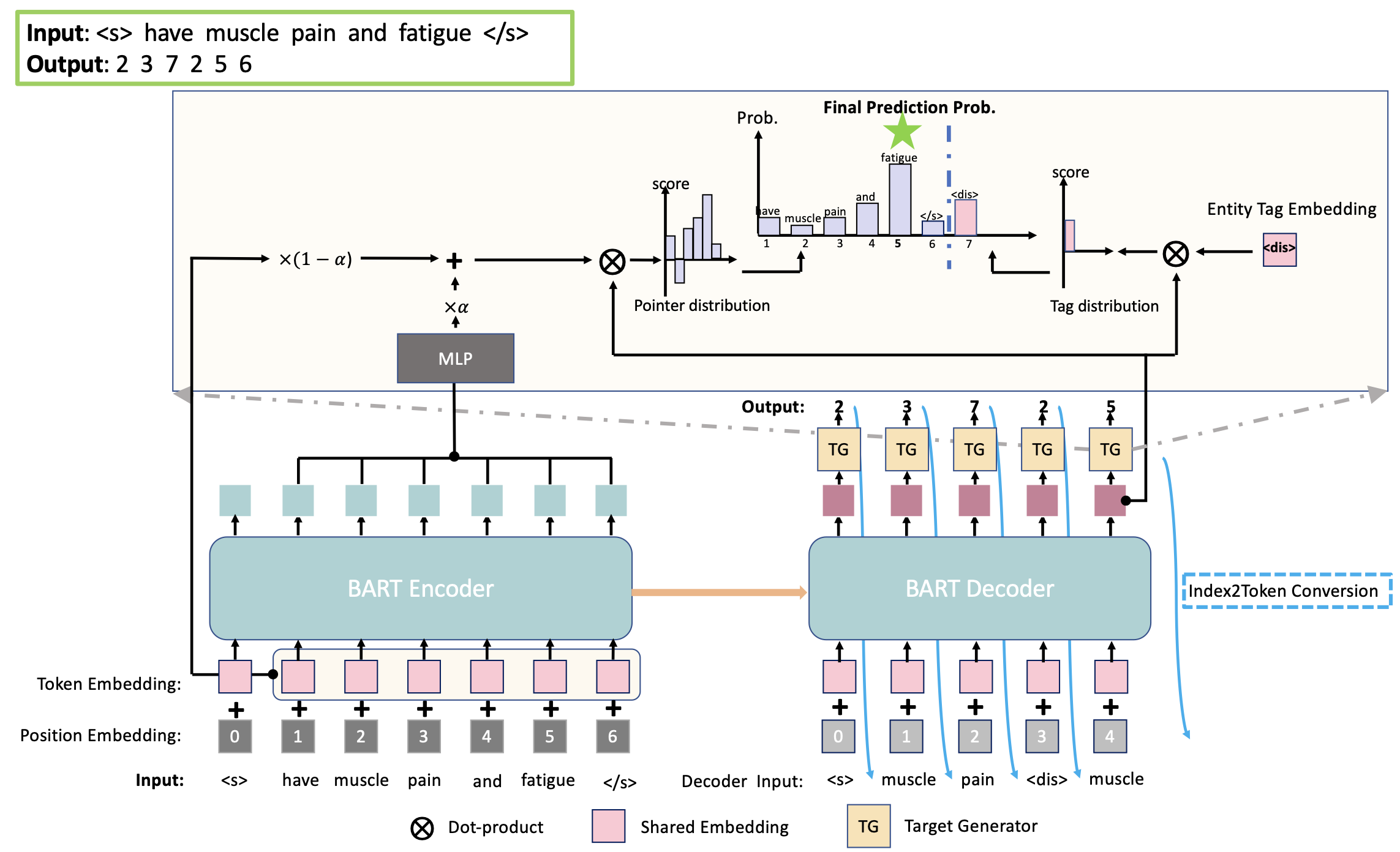## Overview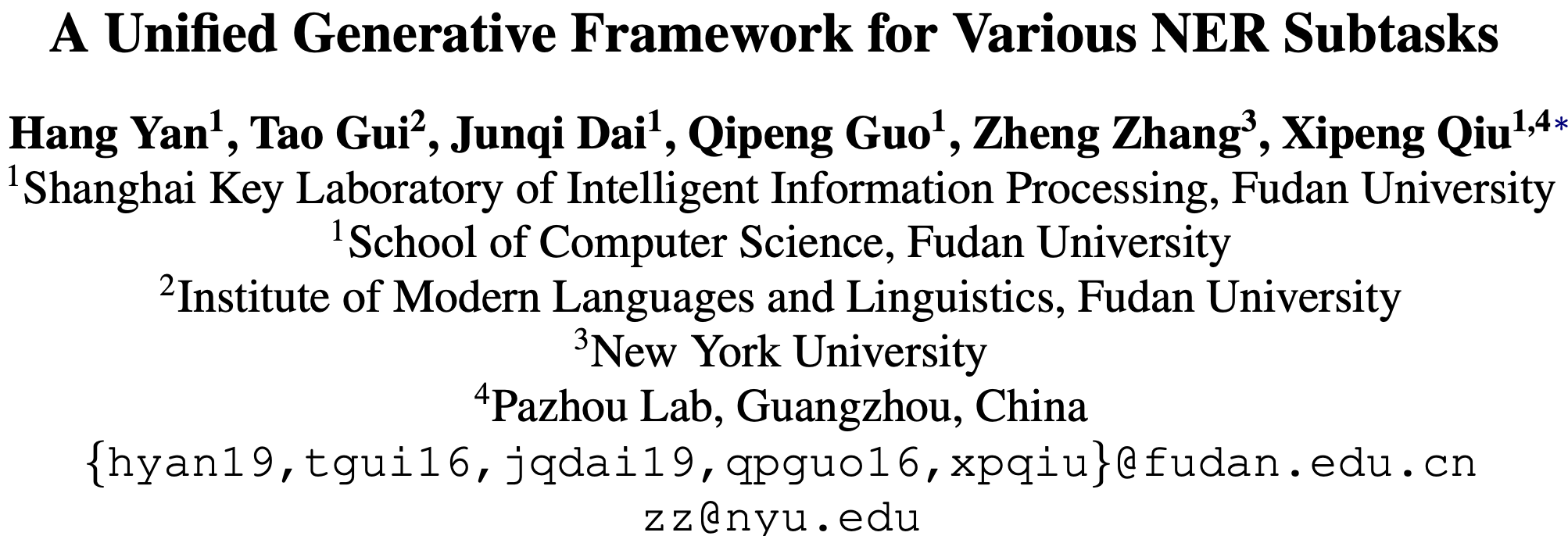## Background

NER旨在识别句子中的实体片段，这些实体可能是嵌套的或者是不连续的，因此NER任务可以分为flat NER、nested NER和discontinuous NER。一般而言，这些子任务可以通过序列标注或者span-level的分类解决。

• 提出了一种生成式的统一框架，将NER问题形式化为实体span序列生成问题。
• 框架融合了预训练模型BART，并提出三种实体表示的序列化方法。
• 提出的框架避免了对标注schema和枚举span的复杂设计，在8个英文数据集上取得了SOTA，包括2个flat NER、3个nested NER和3个discontinuous NER。

## Method

### Notation

• 输入句子$$X=[x_1,x_2,\cdots,x_n]$$
• 目标序列$$Y=[s_{11},e_{11},\cdots,s_{1j},e_{1j},t_1,\cdots,s_{i1},e_{i1},s_{ik},e_{ik},t_i]$$
• 其中$$s$$$$e$$表示一个span的起止下标，$$t_i$$表示标记下标。
• 实体类别$$G=[g_1,\cdots,g_l]$$
• $$l$$表示类别总数
• $$t_i\in(n,n+l]$$，移位是为了不与指针下标混淆。

### Seq2Seq for Unified Decoding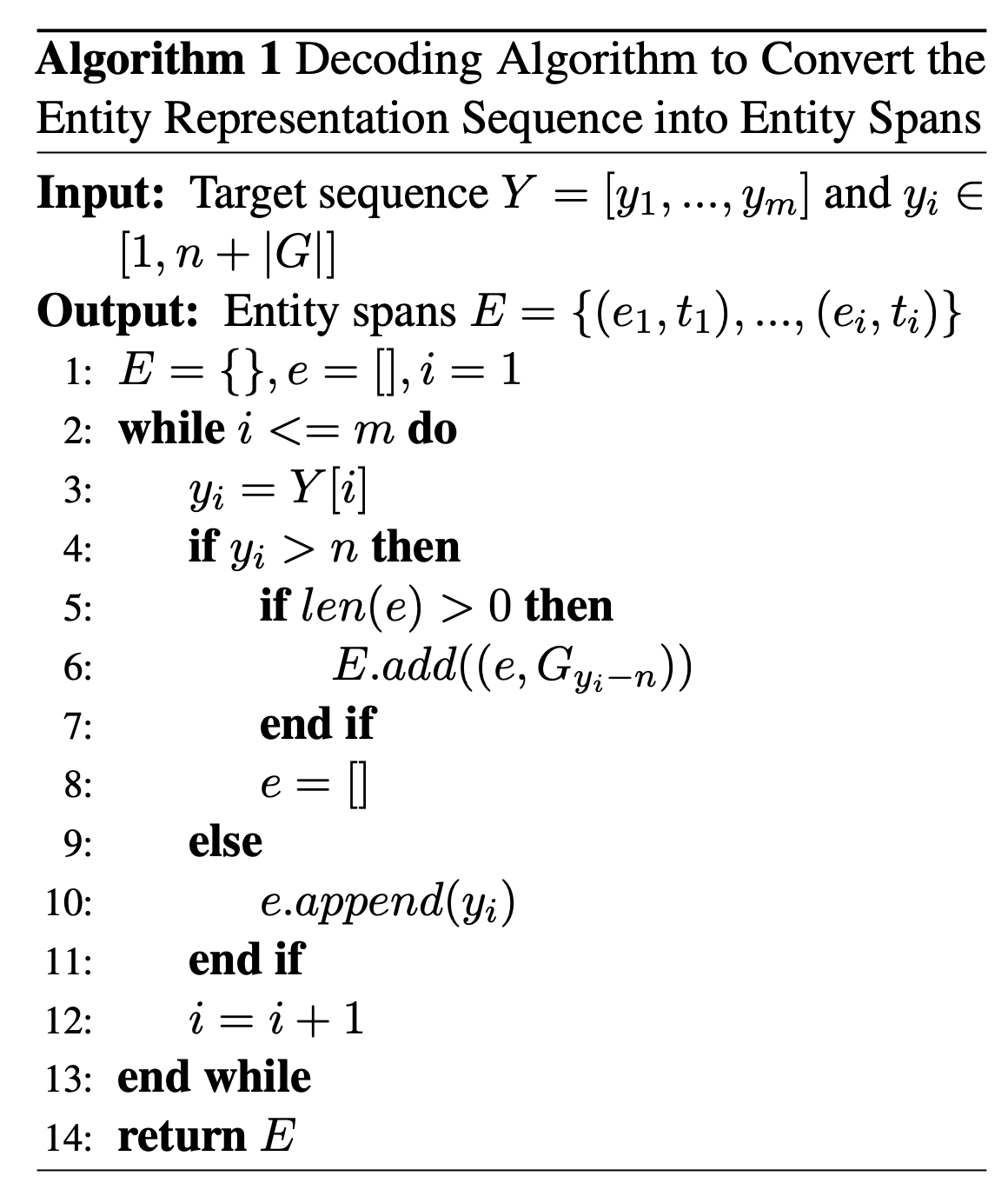BART采用的BPE算法可能会把一个token切分为多个BPE字符，为了更充分利用BART，本文提出了三种基于指针的实体表示，用于定位原始语句中的实体，如下图所示：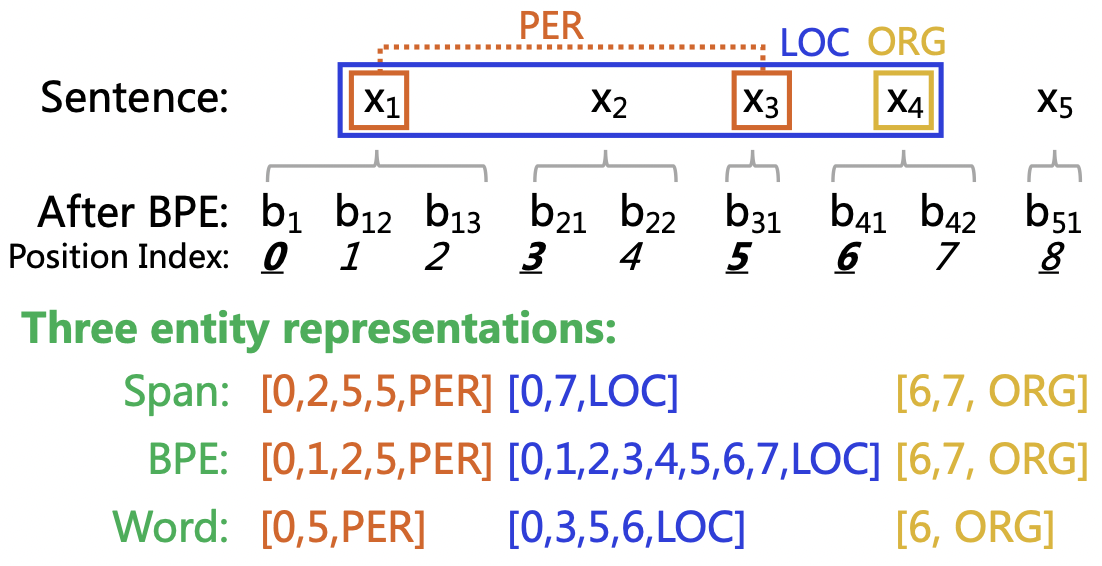• Span：使用实体的起始和截止BPE下标。
• BPE：使用实体的所有BPE下标。
• Word：只使用每个实体的第一个BPE下标。

## Experiment

• Flat NER: CoNLL-2003, OntoNotes
• Nested NER: ACE 2004, ACE 2005, Genia
• Discontinuous NER: CADEC, ShARe13, ShARe14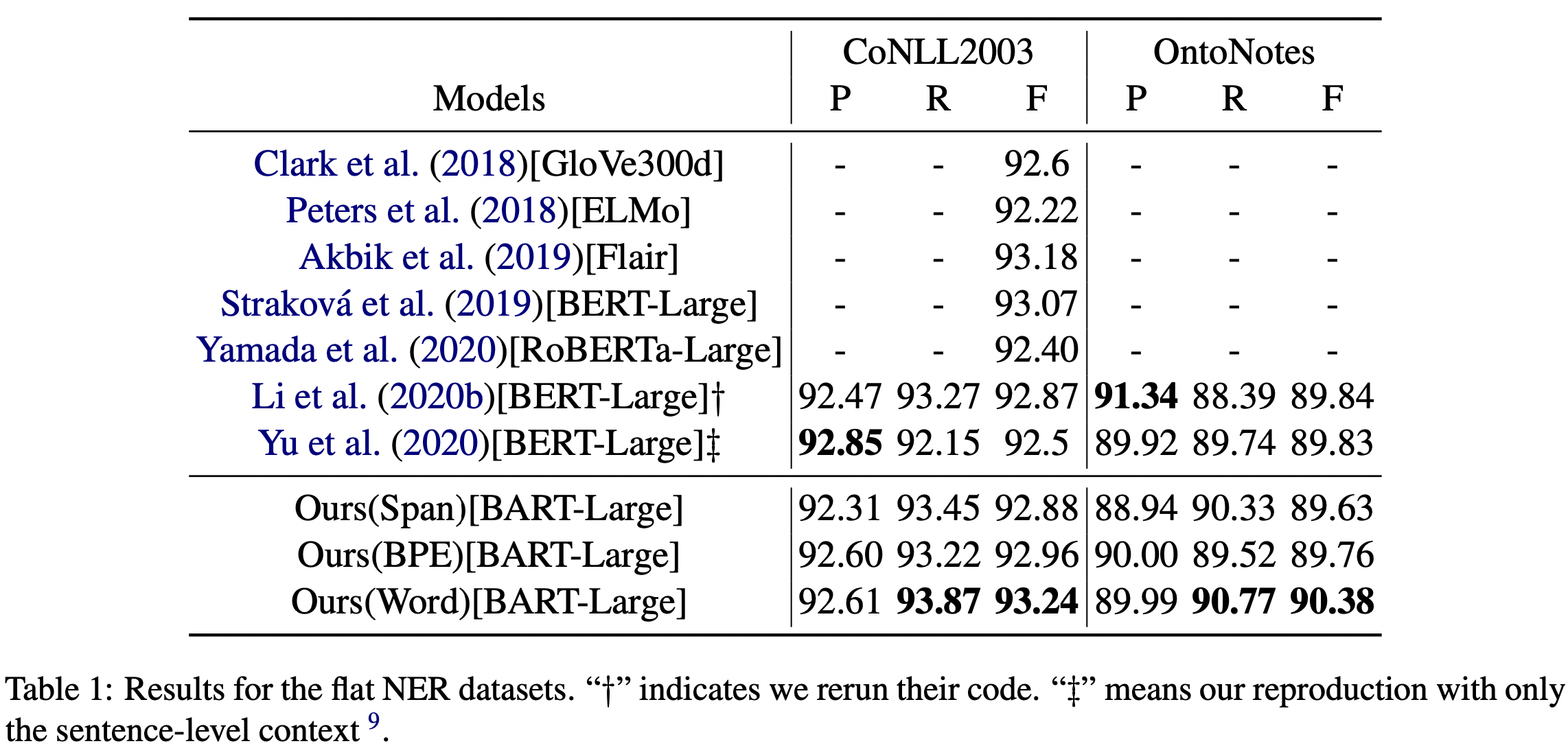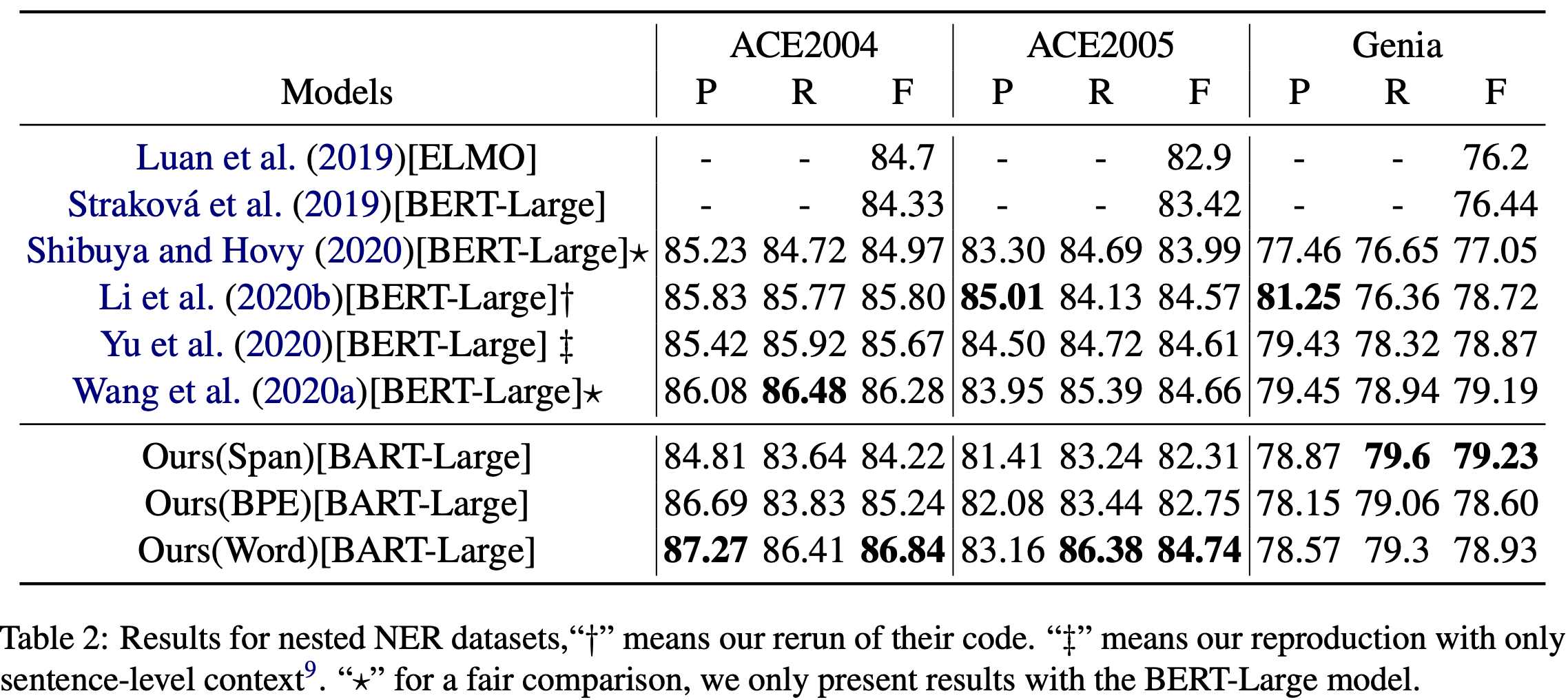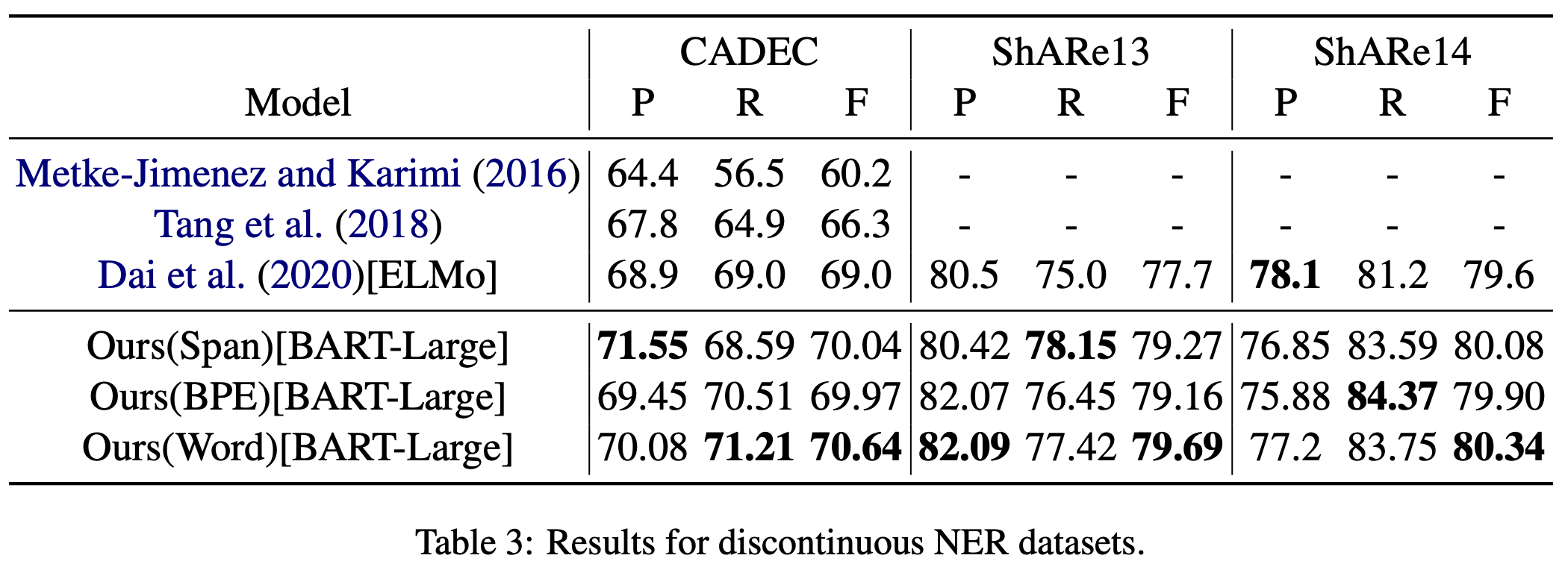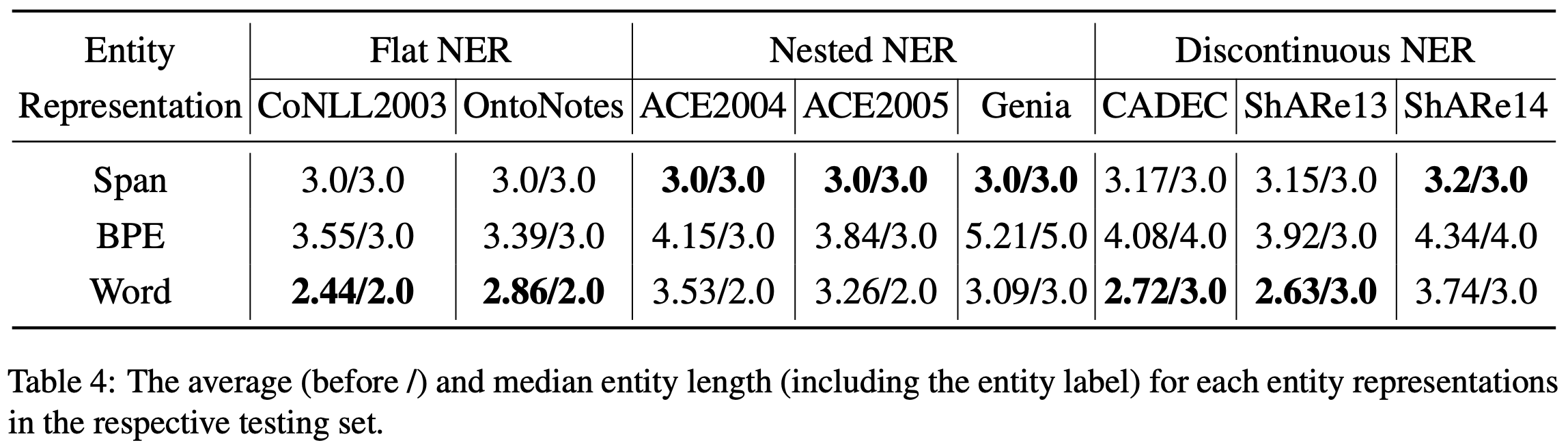Word实体表示比其他两种更好。